Publicité

# New chm 151_unit_9_power_points

27 Feb 2014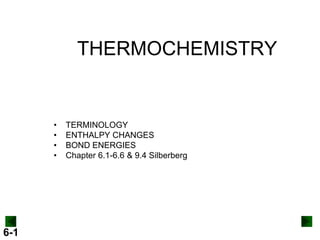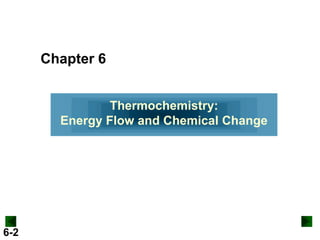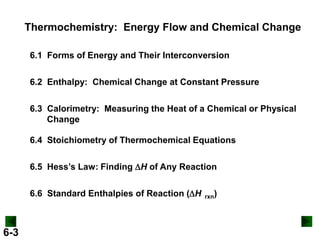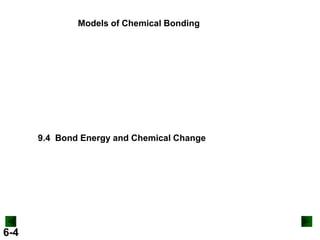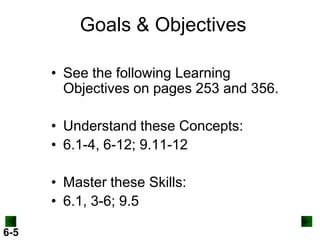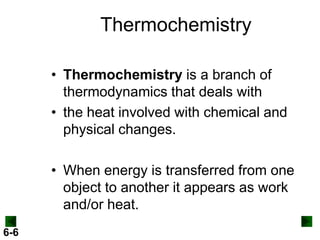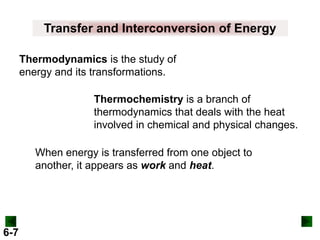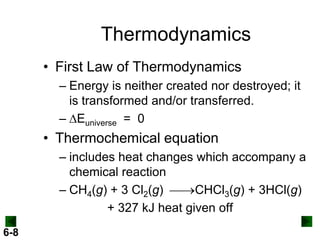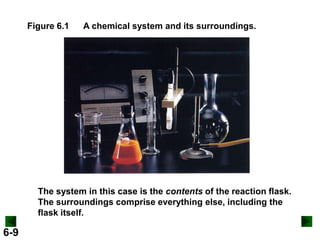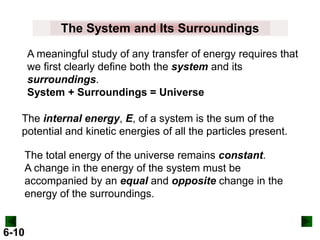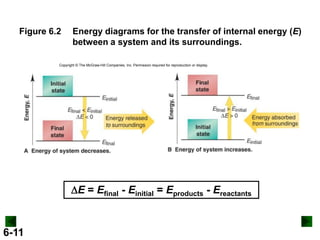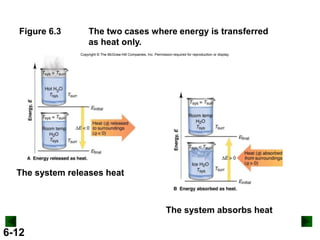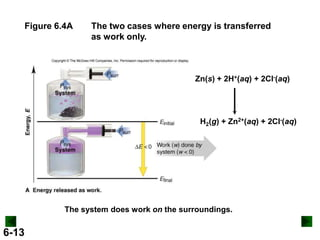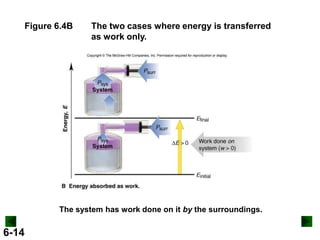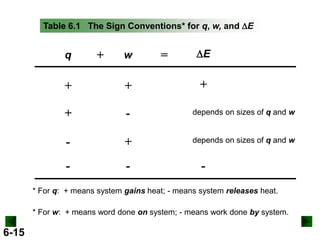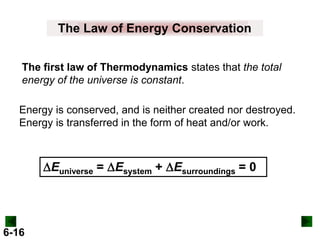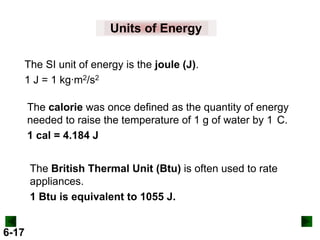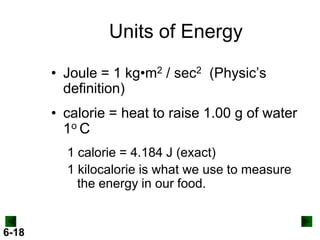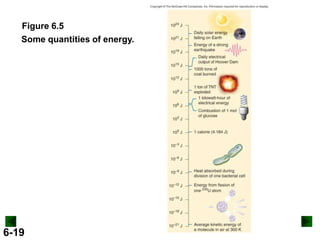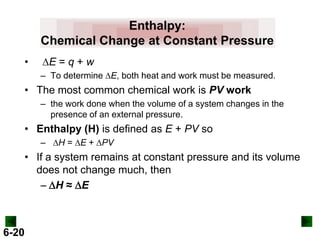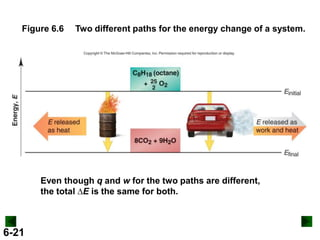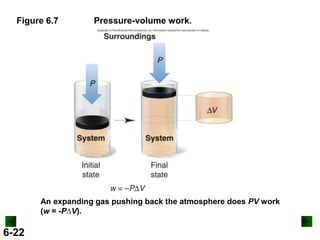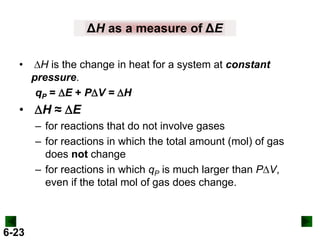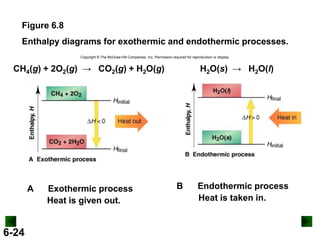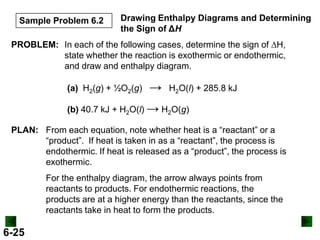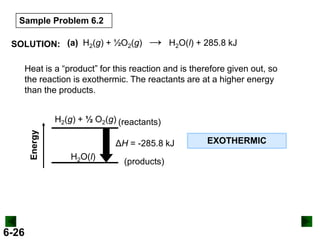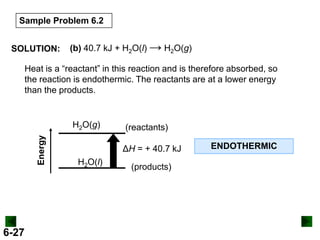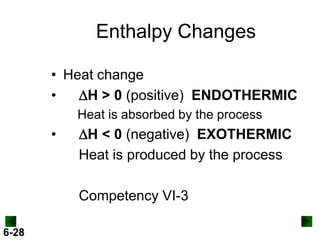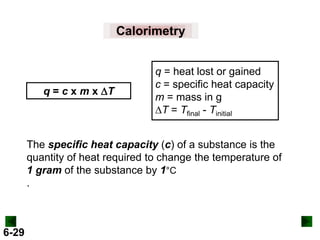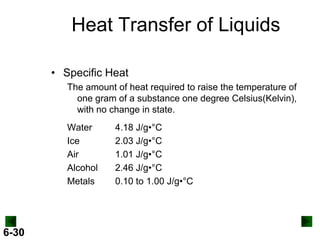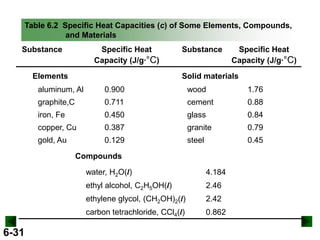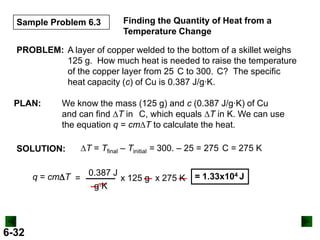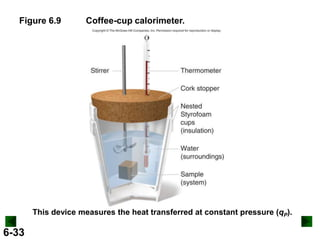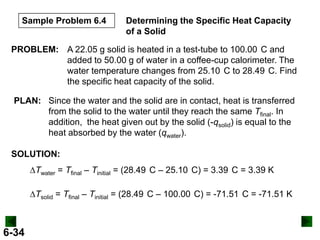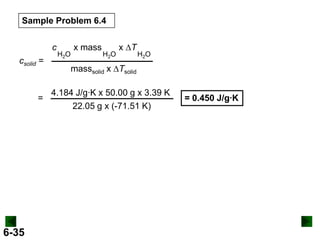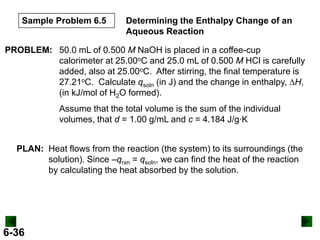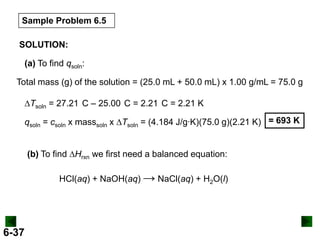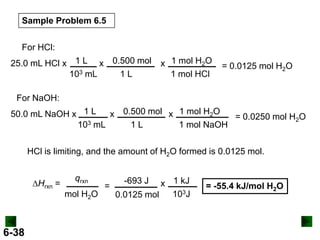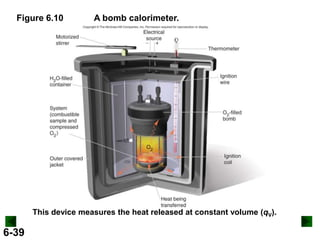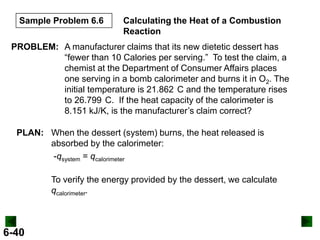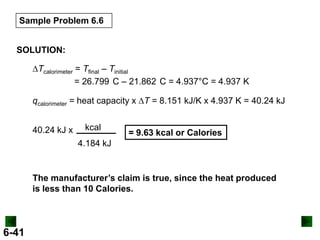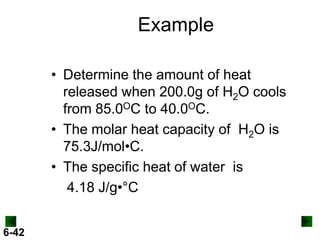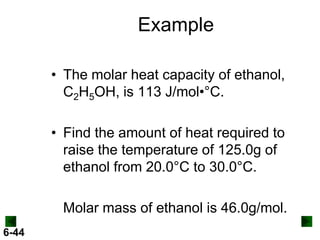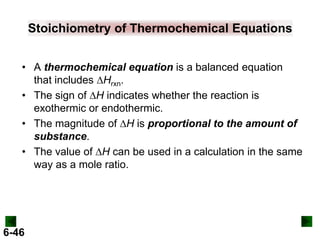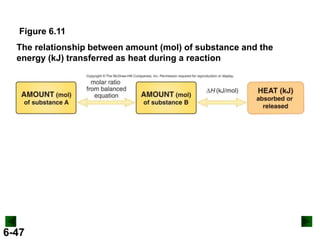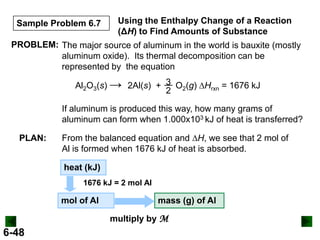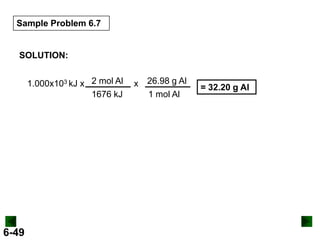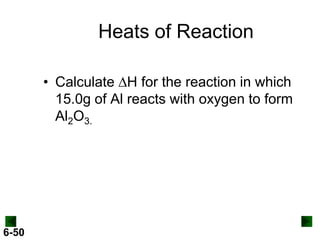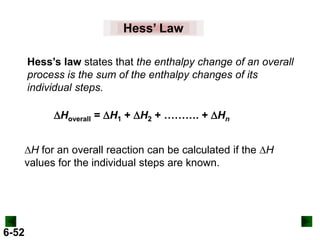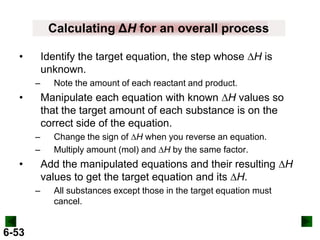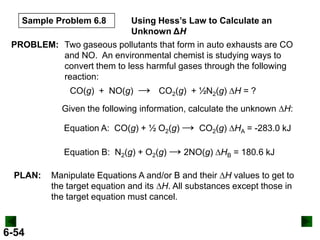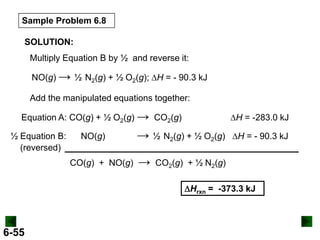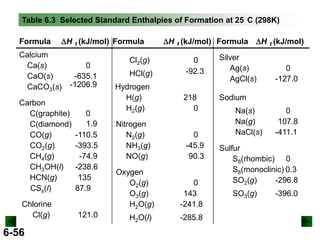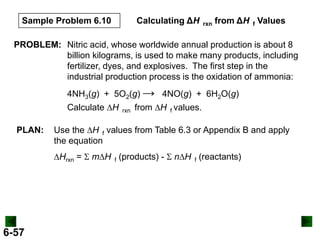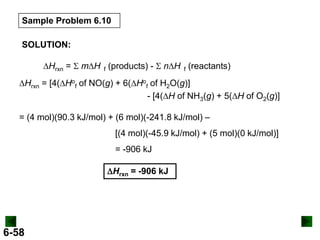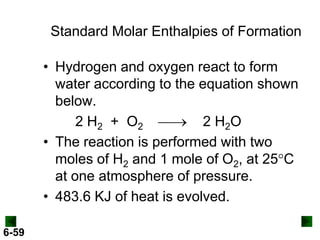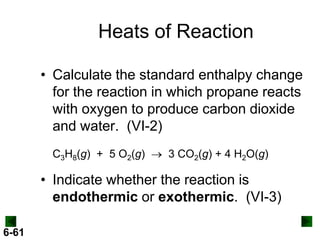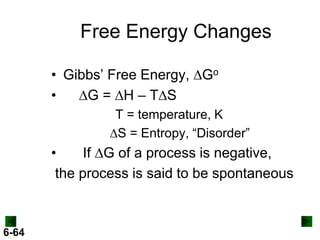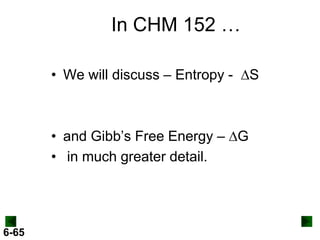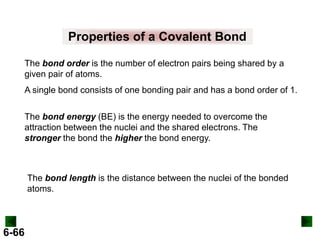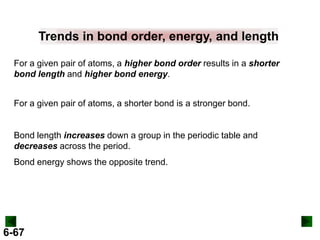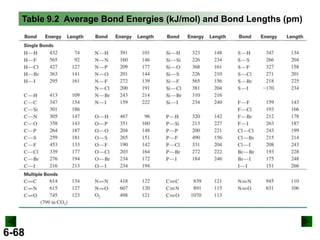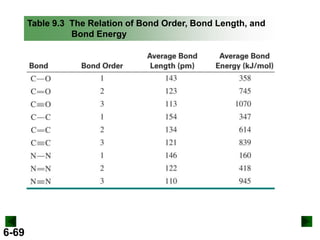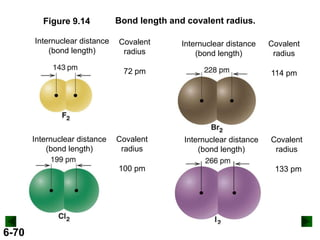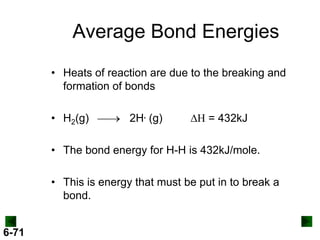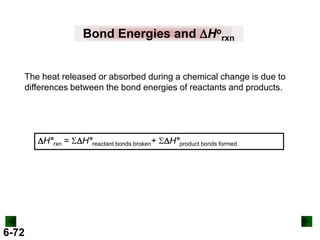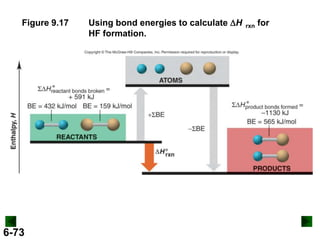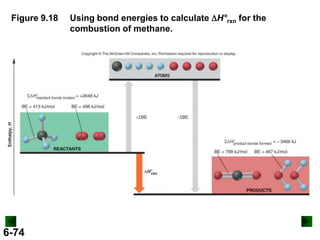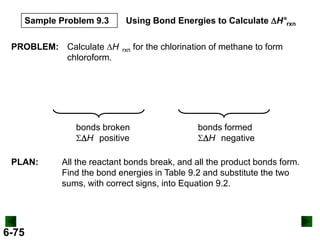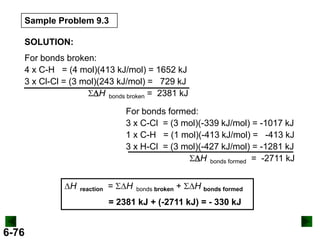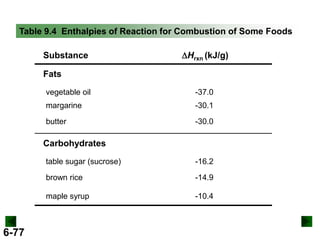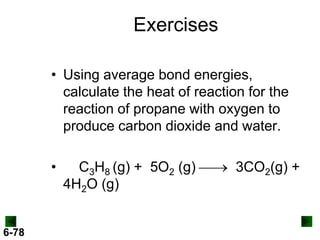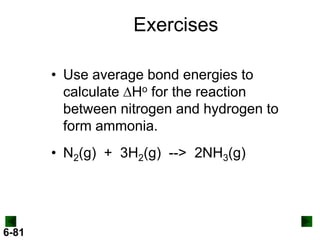1 sur 83
Publicité

### New chm 151_unit_9_power_points

1. THERMOCHEMISTRY • • • • 6-1 TERMINOLOGY ENTHALPY CHANGES BOND ENERGIES Chapter 6.1-6.6 & 9.4 Silberberg
2. Chapter 6 Thermochemistry: Energy Flow and Chemical Change 6-2
3. Thermochemistry: Energy Flow and Chemical Change 6.1 Forms of Energy and Their Interconversion 6.2 Enthalpy: Chemical Change at Constant Pressure 6.3 Calorimetry: Measuring the Heat of a Chemical or Physical Change 6.4 Stoichiometry of Thermochemical Equations 6.5 Hess’s Law: Finding DH of Any Reaction 6.6 Standard Enthalpies of Reaction (DH 6-3 rxn)
4. Models of Chemical Bonding 9.4 Bond Energy and Chemical Change 6-4
5. Goals & Objectives • See the following Learning Objectives on pages 253 and 356. • Understand these Concepts: • 6.1-4, 6-12; 9.11-12 • Master these Skills: • 6.1, 3-6; 9.5 6-5
6. Thermochemistry • Thermochemistry is a branch of thermodynamics that deals with • the heat involved with chemical and physical changes. • When energy is transferred from one object to another it appears as work and/or heat. 6-6
7. Transfer and Interconversion of Energy Thermodynamics is the study of energy and its transformations. Thermochemistry is a branch of thermodynamics that deals with the heat involved in chemical and physical changes. When energy is transferred from one object to another, it appears as work and heat. 6-7
8. Thermodynamics • First Law of Thermodynamics – Energy is neither created nor destroyed; it is transformed and/or transferred. – DEuniverse = 0 • Thermochemical equation – includes heat changes which accompany a chemical reaction – CH4(g) + 3 Cl2(g) CHCl3(g) + 3HCl(g) + 327 kJ heat given off 6-8
9. Figure 6.1 A chemical system and its surroundings. The system in this case is the contents of the reaction flask. The surroundings comprise everything else, including the flask itself. 6-9
10. The System and Its Surroundings A meaningful study of any transfer of energy requires that we first clearly define both the system and its surroundings. System + Surroundings = Universe The internal energy, E, of a system is the sum of the potential and kinetic energies of all the particles present. The total energy of the universe remains constant. A change in the energy of the system must be accompanied by an equal and opposite change in the energy of the surroundings. 6-10
11. Figure 6.2 Energy diagrams for the transfer of internal energy (E) between a system and its surroundings. Copyright © The McGraw-Hill Companies, Inc. Permission required for reproduction or display. DE = Efinal - Einitial = Eproducts - Ereactants 6-11
12. Figure 6.3 The two cases where energy is transferred as heat only. Copyright © The McGraw-Hill Companies, Inc. Permission required for reproduction or display. The system releases heat The system absorbs heat 6-12
13. Figure 6.4A The two cases where energy is transferred as work only. Zn(s) + 2H+(aq) + 2Cl-(aq) H2(g) + Zn2+(aq) + 2Cl-(aq) The system does work on the surroundings. 6-13
14. Figure 6.4B The two cases where energy is transferred as work only. The system has work done on it by the surroundings. 6-14
15. Table 6.1 The Sign Conventions* for q, w, and DE q + w = DE + + + + - depends on sizes of q and w - + depends on sizes of q and w - - - * For q: + means system gains heat; - means system releases heat. * For w: + means word done on system; - means work done by system. 6-15
16. The Law of Energy Conservation The first law of Thermodynamics states that the total energy of the universe is constant. Energy is conserved, and is neither created nor destroyed. Energy is transferred in the form of heat and/or work. DEuniverse = DEsystem + DEsurroundings = 0 6-16
17. Units of Energy The SI unit of energy is the joule (J). 1 J = 1 kg∙m2/s2 The calorie was once defined as the quantity of energy needed to raise the temperature of 1 g of water by 1 C. 1 cal = 4.184 J The British Thermal Unit (Btu) is often used to rate appliances. 1 Btu is equivalent to 1055 J. 6-17
18. Units of Energy • Joule = 1 kg•m2 / sec2 (Physic’s definition) • calorie = heat to raise 1.00 g of water 1o C 1 calorie = 4.184 J (exact) 1 kilocalorie is what we use to measure the energy in our food. 6-18
19. Figure 6.5 Some quantities of energy. 6-19
20. Enthalpy: Chemical Change at Constant Pressure • DE = q + w – To determine DE, both heat and work must be measured. • The most common chemical work is PV work – the work done when the volume of a system changes in the presence of an external pressure. • Enthalpy (H) is defined as E + PV so – DH = DE + DPV • If a system remains at constant pressure and its volume does not change much, then - DH ≈ DE 6-20
21. Figure 6.6 Two different paths for the energy change of a system. Even though q and w for the two paths are different, the total DE is the same for both. 6-21
22. Figure 6.7 Pressure-volume work. An expanding gas pushing back the atmosphere does PV work (w = -PDV). 6-22
23. ΔH as a measure of ΔE • DH is the change in heat for a system at constant pressure. qP = DE + PDV = DH • DH ≈ DE – for reactions that do not involve gases – for reactions in which the total amount (mol) of gas does not change – for reactions in which qP is much larger than PDV, even if the total mol of gas does change. 6-23
24. Figure 6.8 Enthalpy diagrams for exothermic and endothermic processes. Copyright © The McGraw-Hill Companies, Inc. Permission required for reproduction or display. CH4(g) + 2O2(g) → CO2(g) + H2O(g) A 6-24 Exothermic process Heat is given out. H2O(s) → H2O(l) B Endothermic process Heat is taken in.
25. Sample Problem 6.2 Drawing Enthalpy Diagrams and Determining the Sign of ΔH PROBLEM: In each of the following cases, determine the sign of DH, state whether the reaction is exothermic or endothermic, and draw and enthalpy diagram. (a) H2(g) + ½O2(g) → H2O(l) + 285.8 kJ (b) 40.7 kJ + H2O(l) → H2O(g) PLAN: From each equation, note whether heat is a “reactant” or a “product”. If heat is taken in as a “reactant”, the process is endothermic. If heat is released as a “product”, the process is exothermic. For the enthalpy diagram, the arrow always points from reactants to products. For endothermic reactions, the products are at a higher energy than the reactants, since the reactants take in heat to form the products. 6-25
26. Sample Problem 6.2 SOLUTION: (a) H2(g) + ½O2(g) → H2O(l) + 285.8 kJ Heat is a “product” for this reaction and is therefore given out, so the reaction is exothermic. The reactants are at a higher energy than the products. Energy H2(g) + ½ O2(g) (reactants) 6-26 ΔH = -285.8 kJ H2O(l) (products) EXOTHERMIC
27. Sample Problem 6.2 SOLUTION: (b) 40.7 kJ + H2O(l) → H2O(g) Heat is a “reactant” in this reaction and is therefore absorbed, so the reaction is endothermic. The reactants are at a lower energy than the products. Energy H2O(g) 6-27 (reactants) ΔH = + 40.7 kJ H2O(l) (products) ENDOTHERMIC
28. Enthalpy Changes • Heat change • DH > 0 (positive) ENDOTHERMIC Heat is absorbed by the process • DH < 0 (negative) EXOTHERMIC Heat is produced by the process Competency VI-3 6-28
29. Calorimetry q = c x m x DT q = heat lost or gained c = specific heat capacity m = mass in g DT = Tfinal - Tinitial The specific heat capacity (c) of a substance is the quantity of heat required to change the temperature of 1 gram of the substance by 1°C . 6-29
30. Heat Transfer of Liquids • Specific Heat The amount of heat required to raise the temperature of one gram of a substance one degree Celsius(Kelvin), with no change in state. Water Ice Air Alcohol Metals 6-30 4.18 J/g•°C 2.03 J/g•°C 1.01 J/g•°C 2.46 J/g•°C 0.10 to 1.00 J/g•°C
31. Table 6.2 Specific Heat Capacities (c) of Some Elements, Compounds, and Materials Substance Specific Heat Capacity (J/g∙°C) Elements Substance Specific Heat Capacity (J/g∙°C) Solid materials aluminum, Al 0.900 wood 1.76 graphite,C 0.711 cement 0.88 iron, Fe 0.450 glass 0.84 copper, Cu 0.387 granite 0.79 gold, Au 0.129 steel 0.45 Compounds water, H2O(l) ethyl alcohol, C2H5OH(l) 2.46 ethylene glycol, (CH2OH)2(l) 2.42 carbon tetrachloride, CCl4(l) 6-31 4.184 0.862
32. Sample Problem 6.3 Finding the Quantity of Heat from a Temperature Change PROBLEM: A layer of copper welded to the bottom of a skillet weighs 125 g. How much heat is needed to raise the temperature of the copper layer from 25 C to 300. C? The specific heat capacity (c) of Cu is 0.387 J/g∙K. PLAN: We know the mass (125 g) and c (0.387 J/g∙K) of Cu and can find DT in C, which equals DT in K. We can use the equation q = cmDT to calculate the heat. SOLUTION: DT = Tfinal – Tinitial = 300. – 25 = 275 C = 275 K q = cmDT = 0.387 J x 125 g x 275 K g∙K 6-32 = 1.33x104 J
33. Figure 6.9 Coffee-cup calorimeter. This device measures the heat transferred at constant pressure (qP). 6-33
34. Sample Problem 6.4 Determining the Specific Heat Capacity of a Solid PROBLEM: A 22.05 g solid is heated in a test-tube to 100.00 C and added to 50.00 g of water in a coffee-cup calorimeter. The water temperature changes from 25.10 C to 28.49 C. Find the specific heat capacity of the solid. PLAN: Since the water and the solid are in contact, heat is transferred from the solid to the water until they reach the same Tfinal. In addition, the heat given out by the solid (-qsolid) is equal to the heat absorbed by the water (qwater). SOLUTION: DTwater = Tfinal – Tinitial = (28.49 C – 25.10 C) = 3.39 C = 3.39 K DTsolid = Tfinal – Tinitial = (28.49 C – 100.00 C) = -71.51 C = -71.51 K 6-34
35. Sample Problem 6.4 c csolid = = 6-35 H2O x mass H2O x DT H2O masssolid x DTsolid 4.184 J/g∙K x 50.00 g x 3.39 K 22.05 g x (-71.51 K) = 0.450 J/g∙K
36. Sample Problem 6.5 Determining the Enthalpy Change of an Aqueous Reaction PROBLEM: 50.0 mL of 0.500 M NaOH is placed in a coffee-cup calorimeter at 25.00oC and 25.0 mL of 0.500 M HCl is carefully added, also at 25.00oC. After stirring, the final temperature is 27.21oC. Calculate qsoln (in J) and the change in enthalpy, DH, (in kJ/mol of H2O formed). Assume that the total volume is the sum of the individual volumes, that d = 1.00 g/mL and c = 4.184 J/g∙K PLAN: Heat flows from the reaction (the system) to its surroundings (the solution). Since –qrxn = qsoln, we can find the heat of the reaction by calculating the heat absorbed by the solution. 6-36
37. Sample Problem 6.5 SOLUTION: (a) To find qsoln: Total mass (g) of the solution = (25.0 mL + 50.0 mL) x 1.00 g/mL = 75.0 g DTsoln = 27.21 C – 25.00 C = 2.21 C = 2.21 K qsoln = csoln x masssoln x DTsoln = (4.184 J/g∙K)(75.0 g)(2.21 K) = 693 K (b) To find DHrxn we first need a balanced equation: HCl(aq) + NaOH(aq) → NaCl(aq) + H2O(l) 6-37
38. Sample Problem 6.5 For HCl: 25.0 mL HCl x 1 L x 0.500 mol 103 mL 1L x 1 mol H2O 1 mol HCl = 0.0125 mol H2O For NaOH: 50.0 mL NaOH x 1 L x 0.500 mol x 1 mol H2O = 0.0250 mol H2O 3 mL 10 1L 1 mol NaOH HCl is limiting, and the amount of H2O formed is 0.0125 mol. DHrxn = qrxn mol H2O 6-38 = -693 J x 1 kJ 103J 0.0125 mol = -55.4 kJ/mol H2O
39. Figure 6.10 A bomb calorimeter. This device measures the heat released at constant volume (qV). 6-39
40. Sample Problem 6.6 Calculating the Heat of a Combustion Reaction PROBLEM: A manufacturer claims that its new dietetic dessert has “fewer than 10 Calories per serving.” To test the claim, a chemist at the Department of Consumer Affairs places one serving in a bomb calorimeter and burns it in O2. The initial temperature is 21.862 C and the temperature rises to 26.799 C. If the heat capacity of the calorimeter is 8.151 kJ/K, is the manufacturer’s claim correct? PLAN: When the dessert (system) burns, the heat released is absorbed by the calorimeter: -qsystem = qcalorimeter To verify the energy provided by the dessert, we calculate qcalorimeter. 6-40
41. Sample Problem 6.6 SOLUTION: DTcalorimeter = Tfinal – Tinitial = 26.799 C – 21.862 C = 4.937°C = 4.937 K qcalorimeter = heat capacity x DT = 8.151 kJ/K x 4.937 K = 40.24 kJ 40.24 kJ x kcal = 9.63 kcal or Calories 4.184 kJ The manufacturer’s claim is true, since the heat produced is less than 10 Calories. 6-41
42. Example • Determine the amount of heat released when 200.0g of H2O cools from 85.0OC to 40.0OC. • The molar heat capacity of H2O is 75.3J/mol•C. • The specific heat of water is 4.18 J/g•°C 6-42
43. 6-43
44. Example • The molar heat capacity of ethanol, C2H5OH, is 113 J/mol•°C. • Find the amount of heat required to raise the temperature of 125.0g of ethanol from 20.0°C to 30.0°C. Molar mass of ethanol is 46.0g/mol. 6-44
45. 6-45
46. Stoichiometry of Thermochemical Equations • A thermochemical equation is a balanced equation that includes DHrxn. • The sign of DH indicates whether the reaction is exothermic or endothermic. • The magnitude of DH is proportional to the amount of substance. • The value of DH can be used in a calculation in the same way as a mole ratio. 6-46
47. Figure 6.11 The relationship between amount (mol) of substance and the energy (kJ) transferred as heat during a reaction 6-47
48. Sample Problem 6.7 Using the Enthalpy Change of a Reaction (ΔH) to Find Amounts of Substance PROBLEM: The major source of aluminum in the world is bauxite (mostly aluminum oxide). Its thermal decomposition can be represented by the equation Al2O3(s) → 2Al(s) + 3 O2(g) DHrxn = 1676 kJ 2 If aluminum is produced this way, how many grams of aluminum can form when 1.000x103 kJ of heat is transferred? PLAN: From the balanced equation and DH, we see that 2 mol of Al is formed when 1676 kJ of heat is absorbed. heat (kJ) 1676 kJ = 2 mol Al mol of Al mass (g) of Al multiply by M 6-48
49. Sample Problem 6.7 SOLUTION: 1.000x103 kJ x 2 mol Al 1676 kJ 6-49 x 26.98 g Al 1 mol Al = 32.20 g Al
50. Heats of Reaction • Calculate DH for the reaction in which 15.0g of Al reacts with oxygen to form Al2O3. 6-50
51. 6-51
52. Hess’ Law Hess’s law states that the enthalpy change of an overall process is the sum of the enthalpy changes of its individual steps. DHoverall = DH1 + DH2 + ………. + DHn DH for an overall reaction can be calculated if the DH values for the individual steps are known. 6-52
53. Calculating ΔH for an overall process Identify the target equation, the step whose DH is unknown. • – Manipulate each equation with known DH values so that the target amount of each substance is on the correct side of the equation. • – – Change the sign of DH when you reverse an equation. Multiply amount (mol) and DH by the same factor. Add the manipulated equations and their resulting DH values to get the target equation and its DH. • – 6-53 Note the amount of each reactant and product. All substances except those in the target equation must cancel.
54. Using Hess’s Law to Calculate an Unknown ΔH PROBLEM: Two gaseous pollutants that form in auto exhausts are CO and NO. An environmental chemist is studying ways to convert them to less harmful gases through the following reaction: Sample Problem 6.8 CO(g) + NO(g) → CO2(g) + ½N2(g) DH = ? Given the following information, calculate the unknown DH: Equation A: CO(g) + ½ O2(g) → CO2(g) DHA = -283.0 kJ Equation B: N2(g) + O2(g) → 2NO(g) DHB = 180.6 kJ PLAN: 6-54 Manipulate Equations A and/or B and their DH values to get to the target equation and its DH. All substances except those in the target equation must cancel.
55. Sample Problem 6.8 SOLUTION: Multiply Equation B by ½ and reverse it: NO(g) → ½ N2(g) + ½ O2(g); DH = - 90.3 kJ Add the manipulated equations together: Equation A: CO(g) + ½ O2(g) → CO2(g) ½ Equation B: (reversed) NO(g) CO(g) + NO(g) DH = -283.0 kJ → ½ N2(g) + ½ O2(g) → DH = - 90.3 kJ CO2(g) + ½ N2(g) DHrxn = -373.3 kJ 6-55
56. Table 6.3 Selected Standard Enthalpies of Formation at 25 C (298K) Formula DH f (kJ/mol) Formula Calcium Ca(s) CaO(s) CaCO3(s) 0 -635.1 -1206.9 Carbon C(graphite) C(diamond) CO(g) CO2(g) CH4(g) CH3OH(l) HCN(g) CSs(l) Chlorine Cl(g) 6-56 0 1.9 -110.5 -393.5 -74.9 -238.6 135 87.9 121.0 DH f (kJ/mol) Formula DH f (kJ/mol) 0 -92.3 Silver Ag(s) AgCl(s) Hydrogen H(g) H2(g) 218 0 Sodium Nitrogen N2(g) NH3(g) NO(g) 0 -45.9 90.3 Cl2(g) HCl(g) Oxygen O2(g) O3(g) H2O(g) H2O(l) 0 143 -241.8 -285.8 Na(s) Na(g) NaCl(s) 0 -127.0 0 107.8 -411.1 Sulfur S8(rhombic) 0 S8(monoclinic) 0.3 SO2(g) -296.8 SO3(g) -396.0
57. Calculating ΔH Sample Problem 6.10 rxn from ΔH f Values PROBLEM: Nitric acid, whose worldwide annual production is about 8 billion kilograms, is used to make many products, including fertilizer, dyes, and explosives. The first step in the industrial production process is the oxidation of ammonia: 4NH3(g) + 5O2(g) → 4NO(g) + 6H2O(g) Calculate DH PLAN: rxn from DH f values. Use the DH f values from Table 6.3 or Appendix B and apply the equation DHrxn = S mDH f (products) - S nDH f (reactants) 6-57
58. Sample Problem 6.10 SOLUTION: DHrxn = S mDH f (products) - S nDH f (reactants) DHrxn = [4(DHof of NO(g) + 6(DHof of H2O(g)] - [4(DH of NH3(g) + 5(DH of O2(g)] = (4 mol)(90.3 kJ/mol) + (6 mol)(-241.8 kJ/mol) – [(4 mol)(-45.9 kJ/mol) + (5 mol)(0 kJ/mol)] = -906 kJ DHrxn = -906 kJ 6-58
59. Standard Molar Enthalpies of Formation • Hydrogen and oxygen react to form water according to the equation shown below. 2 H2 + O2  2 H2O • The reaction is performed with two moles of H2 and 1 mole of O2, at 25C at one atmosphere of pressure. • 483.6 KJ of heat is evolved. 6-59
60. 6-60
61. Heats of Reaction • Calculate the standard enthalpy change for the reaction in which propane reacts with oxygen to produce carbon dioxide and water. (VI-2) C3H8(g) + 5 O2(g)  3 CO2(g) + 4 H2O(g) • Indicate whether the reaction is endothermic or exothermic. (VI-3) 6-61
62. 6-62
63. Free Energy Changes • Second Law of Thermodynamics – In a spontaneous process, the entropy of a system and its surroundings increases – DS for the universe > 0 – Think about your room as a kid. 6-63
64. Free Energy Changes • Gibbs’ Free Energy, DGo • DG = DH – TDS T = temperature, K DS = Entropy, “Disorder” • If DG of a process is negative, the process is said to be spontaneous 6-64
65. In CHM 152 … • We will discuss – Entropy - DS • and Gibb’s Free Energy – DG • in much greater detail. 6-65
66. Properties of a Covalent Bond The bond order is the number of electron pairs being shared by a given pair of atoms. A single bond consists of one bonding pair and has a bond order of 1. The bond energy (BE) is the energy needed to overcome the attraction between the nuclei and the shared electrons. The stronger the bond the higher the bond energy. The bond length is the distance between the nuclei of the bonded atoms. 6-66
67. Trends in bond order, energy, and length For a given pair of atoms, a higher bond order results in a shorter bond length and higher bond energy. For a given pair of atoms, a shorter bond is a stronger bond. Bond length increases down a group in the periodic table and decreases across the period. Bond energy shows the opposite trend. 6-67
68. Table 9.2 Average Bond Energies (kJ/mol) and Bond Lengths (pm) 6-68
69. Table 9.3 The Relation of Bond Order, Bond Length, and Bond Energy 6-69
70. Figure 9.14 Internuclear distance (bond length) Bond length and covalent radius. Covalent radius Internuclear distance (bond length) 72 pm Internuclear distance (bond length) Covalent radius 100 pm 6-70 Covalent radius 114 pm Internuclear distance (bond length) Covalent radius 133 pm
71. Average Bond Energies • Heats of reaction are due to the breaking and formation of bonds • H2(g)  2H. (g) DH = 432kJ • The bond energy for H-H is 432kJ/mole. • This is energy that must be put in to break a bond. 6-71
72. Bond Energies and DHorxn The heat released or absorbed during a chemical change is due to differences between the bond energies of reactants and products. DH°rxn = SDH°reactant bonds broken+ SDH°product bonds formed 6-72
73. Figure 9.17 6-73 Using bond energies to calculate DH HF formation. rxn for
74. Figure 9.18 6-74 Using bond energies to calculate DH°rxn for the combustion of methane.
75. Sample Problem 9.3 PROBLEM: Calculate DH chloroform. Using Bond Energies to Calculate DH°rxn rxn bonds broken SDH positive PLAN: 6-75 for the chlorination of methane to form bonds formed SDH negative All the reactant bonds break, and all the product bonds form. Find the bond energies in Table 9.2 and substitute the two sums, with correct signs, into Equation 9.2.
76. Sample Problem 9.3 SOLUTION: For bonds broken: 4 x C-H = (4 mol)(413 kJ/mol) = 1652 kJ 3 x Cl-Cl = (3 mol)(243 kJ/mol) = 729 kJ SDH bonds broken = 2381 kJ For bonds formed: 3 x C-Cl = (3 mol)(-339 kJ/mol) = -1017 kJ 1 x C-H = (1 mol)(-413 kJ/mol) = -413 kJ 3 x H-Cl = (3 mol)(-427 kJ/mol) = -1281 kJ SDH bonds formed = -2711 kJ DH reaction = SDH bonds broken + SDH bonds formed = 2381 kJ + (-2711 kJ) = - 330 kJ 6-76
77. Table 9.4 Enthalpies of Reaction for Combustion of Some Foods Substance DHrxn (kJ/g) Fats vegetable oil -37.0 margarine -30.1 butter -30.0 Carbohydrates table sugar (sucrose) brown rice -14.9 maple syrup 6-77 -16.2 -10.4
78. Exercises • Using average bond energies, calculate the heat of reaction for the reaction of propane with oxygen to produce carbon dioxide and water. • 6-78 C3H8 (g) + 5O2 (g)  3CO2(g) + 4H2O (g)
79. 6-79
80. 6-80
81. Exercises • Use average bond energies to calculate DHo for the reaction between nitrogen and hydrogen to form ammonia. • N2(g) + 3H2(g) --> 2NH3(g) 6-81
82. 6-82
83. 6-83
Publicité